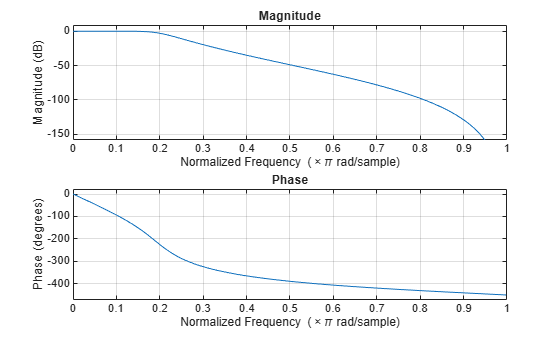# zp2sos

Convert zero-pole-gain filter parameters to second-order sections form

## Syntax

``[sos,g] = zp2sos(z,p,k)``
``[sos,g] = zp2sos(z,p,k,order)``
``[sos,g] = zp2sos(z,p,k,order,scale)``
``[sos,g] = zp2sos(z,p,k,order,scale,zeroflag)``
``sos = zp2sos(___)``

## Description

example

````[sos,g] = zp2sos(z,p,k)` finds a second-order section matrix `sos` with gain `g` that is equivalent to the transfer function H(z) whose n zeros, m poles, and scalar gain are specified in `z`, `p`, and `k`: $H\left(z\right)=k\frac{\left(z-{z}_{1}\right)\left(z-{z}_{2}\right)\cdots \left(z-{z}_{n}\right)}{\left(z-{p}_{1}\right)\left(z-{p}_{2}\right)\cdots \left(z-{p}_{m}\right)}.$```
````[sos,g] = zp2sos(z,p,k,order)` specifies the order of the rows in `sos`.```
````[sos,g] = zp2sos(z,p,k,order,scale)` specifies the scaling of the gain and numerator coefficients of all second-order sections.```
````[sos,g] = zp2sos(z,p,k,order,scale,zeroflag)` specifies the handling of real zeros that are negatives of each other.```
````sos = zp2sos(___)` embeds the overall system gain in the first section.```

## Examples

collapse all

Design a 5th-order Butterworth lowpass filter using the function `butter` with output expressed in zero-pole-gain form. Specify the cutoff frequency to be one-fifth of the Nyquist frequency. Convert the result to second-order sections. Visualize the magnitude response.

```[z,p,k] = butter(5,0.2); sos = zp2sos(z,p,k)```
```sos = 3×6 0.0013 0.0013 0 1.0000 -0.5095 0 1.0000 2.0000 1.0000 1.0000 -1.0966 0.3554 1.0000 2.0000 1.0000 1.0000 -1.3693 0.6926 ```
`fvtool(sos)`## Input Arguments

collapse all

Zeros of the system, specified as a vector. The zeros must be real or come in complex conjugate pairs.

Example: `[1 (1+1j)/2 (1-1j)/2]'`

Data Types: `double`
Complex Number Support: Yes

Poles of the system, specified as a vector. The poles must be real or come in complex conjugate pairs.

Example: `[1 (1+1j)/2 (1-1j)/2]'`

Data Types: `double`
Complex Number Support: Yes

Scalar gain of the system, specified as a scalar.

Data Types: `double`

Row order, specified as one of the following:

• `'up'` — Order the sections so the first row of `sos` contains the poles farthest from the unit circle.

• `'down'` — Order the sections so the first row of `sos` contains the poles closest to the unit circle.

Data Types: `char`

Scaling of gain and numerator coefficients, specified as one of the following:

• `'none'` — Apply no scaling.

• `'inf'` — Apply infinity-norm scaling.

• `'two'` — Apply 2-norm scaling.

Using infinity-norm scaling with `'up'`-ordering minimizes the probability of overflow in the realization. Using 2-norm scaling with `'down'`-ordering minimizes the peak round-off noise.

Note

Infinity-norm and 2-norm scaling are appropriate only for direct-form II implementations.

Data Types: `char`

Ordering of real zeros that are negatives of each other, specified as a logical scalar.

• If you specify `zeroflag` as `false`, the function orders those zeros according to proximity to poles.

• If you specify `zeroflag` as `true`, the function keeps those zeros together. This option results in a numerator with a middle coefficient equal to zero.

Data Types: `logical`

## Output Arguments

collapse all

Second-order section representation, returned as a matrix. `sos` is an L-by-6 matrix

`$\text{sos}=\left[\begin{array}{cccccc}{b}_{01}& {b}_{11}& {b}_{21}& 1& {a}_{11}& {a}_{21}\\ {b}_{02}& {b}_{12}& {b}_{22}& 1& {a}_{12}& {a}_{22}\\ ⋮& ⋮& ⋮& ⋮& ⋮& ⋮\\ {b}_{0L}& {b}_{1L}& {b}_{2L}& 1& {a}_{1L}& {a}_{2L}\end{array}\right]$`

whose rows contain the numerator and denominator coefficients bik and aik of the second-order sections of H(z):

`$H\left(z\right)=g\prod _{k=1}^{L}{H}_{k}\left(z\right)=g\prod _{k=1}^{L}\frac{{b}_{0k}+{b}_{1k}{z}^{-1}+{b}_{2k}{z}^{-2}}{1+{a}_{1k}{z}^{-1}+{a}_{2k}{z}^{-2}}.$`

If the transfer function has n zeros and m poles, then L is the closest integer greater than or equal to max(n/2,m/2).

Overall system gain, returned as a real scalar.

If you call `zp2sos` with one output argument, the function embeds the overall system gain in the first section, H1(z), so that

`$H\left(z\right)=\prod _{k=1}^{L}{H}_{k}\left(z\right).$`

Note

Embedding the gain in the first section when scaling a direct-form II structure is not recommended and can result in erratic scaling. To avoid embedding the gain, use `zp2sos` with two outputs.

## Algorithms

`zp2sos` uses a four-step algorithm to determine the second-order section representation for an input zero-pole-gain system:

1. It groups the zeros and poles into complex conjugate pairs using the `cplxpair` function.

2. It forms the second-order section by matching the pole and zero pairs according to the following rules:

1. Match the poles closest to the unit circle with the zeros closest to those poles.

2. Match the poles next closest to the unit circle with the zeros closest to those poles.

3. Continue until all of the poles and zeros are matched.

`zp2sos` groups real poles into sections with the real poles closest to them in absolute value. The same rule holds for real zeros.

3. It orders the sections according to the proximity of the pole pairs to the unit circle. `zp2sos` normally orders the sections with poles closest to the unit circle last in the cascade. You can tell `zp2sos` to order the sections in the reverse order using the `order` argument.

4. `zp2sos` scales the sections by the norm specified in `scale`. For arbitrary H(ω), the scaling is defined by

`${‖H‖}_{p}={\left[\frac{1}{2\pi }\underset{0}{\overset{2\pi }{\int }}{|H\left(\omega \right)|}^{p}d\omega \right]}^{1/p}$`

where p can be either infinity or 2. This scaling is an attempt to minimize overflow or peak round-off noise in fixed-point filter implementations.

 Jackson, L. B. Digital Filters and Signal Processing. 3rd ed. Boston: Kluwer Academic Publishers, 1996.

 Mitra, Sanjit Kumar. Digital Signal Processing: A Computer-Based Approach. 3rd ed. New York: McGraw-Hill Higher Education, 2006.

 Vaidyanathan, P. P. "Robust Digital Filter Structures." Handbook for Digital Signal Processing (S. K. Mitra and J. F. Kaiser, eds.). New York: John Wiley & Sons, 1993.